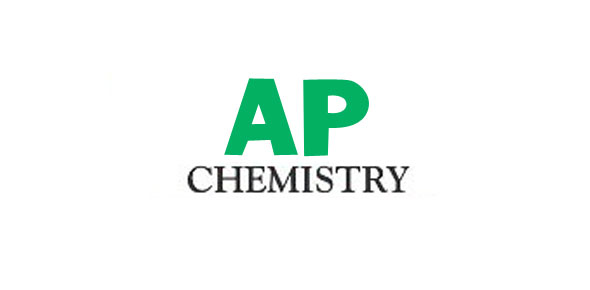# AP Chemistry Chapter 2 Quiz (Part 1)

11 Questions | Total Attempts: 396SettingsThis quiz covers sections 2. 1-2. 5 in Chapter 2: Atoms, Molecules, and Ions in the textbook.

• 1.
Who first used the term "atom" to describe small, invisible particles?
• A.

Boyle

• B.

Lavoisier

• C.

Democritus

• D.

Bauer

• 2.
Who verified a law that suggested mass is neither created nor destroyed?
• A.

Dalton

• B.

Lavoisier

• C.

Stahl

• D.

Bauer

• 3.
The principle of the constant composition of compounds is called what?
• A.

Law of conservation of mass

• B.

Law of definite proportions

• C.

Law of conservation of energy

• D.

Law of multiple proportions

• 4.
When two elements form a series of compounds, the ratios of masses of the second element that combine with 1 gram of the first element can always be reduced to small whole numbers is called ____________?
• A.

Law of definite proportions

• B.

Law of conservation of mass

• C.

Law of multiple proportions

• D.

Law of conservation of energy

• 5.
Which is NOT part of Dalton's Atomic Theory?
• A.

Each element is made up of tiny particles called atoms

• B.

The atoms of a given element are identical; the atoms of different elements are different in some fundamental way or ways.

• C.

Mass of atoms is neither created nor destroyed

• D.

Chemical compounds are formed when atoms of different elements combine with each other. A given compound always has the same relative numbers and types of atoms.

• 6.
Negatively charged particles are called ___________.
• A.

Quarks

• B.

Protons

• C.

Neutrons

• D.

Electrons

• 7.
An atom that consists of a diffuse cloud of positive charge with the negative electrons embedded randomly in it is a description of what 20th century model?
• A.

• B.

Atomus

• C.

Oil-drop experiment

• D.

Plum pudding model

• 8.
Which is not a type of radioactive emission?
• A.

Beta

• B.

Atomic

• C.

Gamma

• D.

Alpha

• 9.
Atoms with the same number of protons but different numbers of neutrons are called what?
• A.

Protons

• B.

• C.

Nuclear atoms

• D.

Isotopes

• 10.
What is the mass number of Fluorine?
• A.

19

• B.

10

• C.

9

• D.

0

• 11.
How many protons are in an atom of Sodium?
• A.

23

• B.

11

• C.

12

• D.

0

Related TopicsBack to top